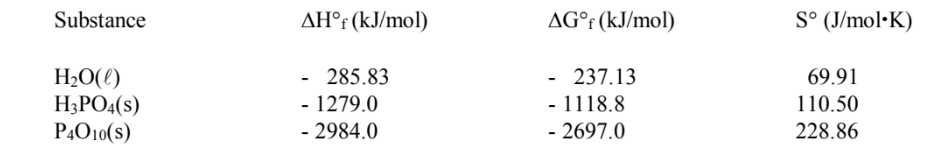Problem: Thermodynamic data are given below (at T = 25. °C) and may be of use in doing this problem.Phosphoric acid (H3PO4) may be prepared by adding tetraphosphorus decaoxide (P4O10) to water (H2O), by the process                P 4O10(s) + 6 H2O(I) → 4 H3PO4(s)a) What are ΔS°rxn and ΔG°rxn for the below reaction, at T = 25.0 °C?b) Give the correctly balanced formation reaction for H 3PO4(s).

FREE Expert Solution
83% (123 ratings)
Problem Details

Thermodynamic data are given below (at T = 25. °C) and may be of use in doing this problem.

Phosphoric acid (H3PO4) may be prepared by adding tetraphosphorus decaoxide (P4O10) to water (H2O), by the process

P 4O10(s) + 6 H2O(I) → 4 H3PO4(s)

a) What are ΔS°rxn and ΔG°rxn for the below reaction, at T = 25.0 °C?

b) Give the correctly balanced formation reaction for H 3PO4(s).What scientific concept do you need to know in order to solve this problem?

Our tutors have indicated that to solve this problem you will need to apply the Gibbs Free Energy concept. You can view video lessons to learn Gibbs Free Energy Or if you need more Gibbs Free Energy practice, you can also practice Gibbs Free Energy practice problems .

What is the difficulty of this problem?

Our tutors rated the difficulty of Thermodynamic data are given below (at T = 25. °C) and may b... as high difficulty.

How long does this problem take to solve?

Our expert Chemistry tutor, Dasha took 11 minutes to solve this problem. You can follow their steps in the video explanation above.

What professor is this problem relevant for?

Based on our data, we think this problem is relevant for Professor Roychowdhury's class at RUTGERS.